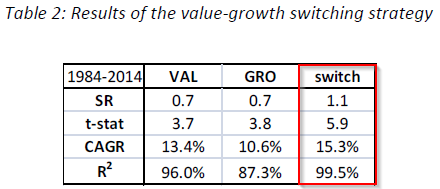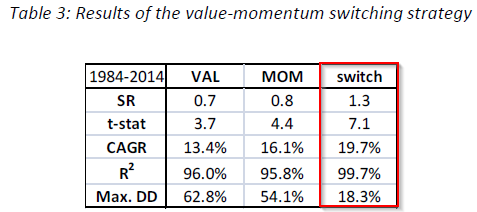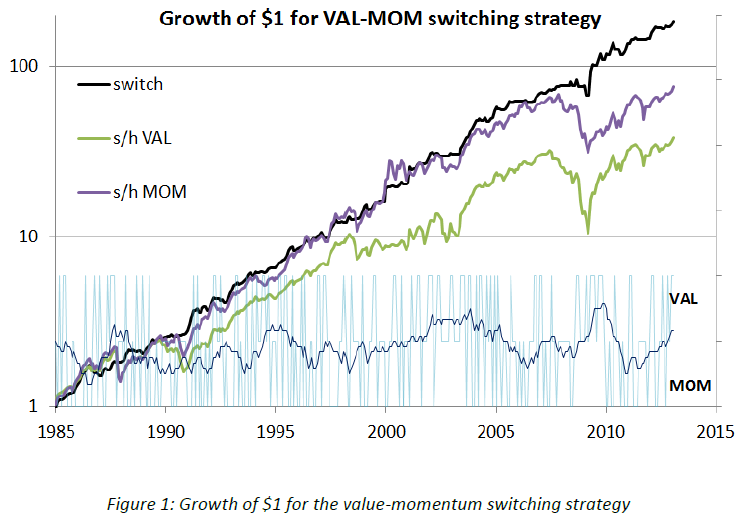### Remark:

The Fama-French three factor model is ubiquitous in modern finance. Returns are modeled as a linear combination of a market factor, a size factor and a book-to-market equity ratio (or “value”) factor. The success of this approach, since its introduction in 1992, has resulted in widespread adoption and a large body of related academic literature. The risk factors exhibit serial correlation at a monthly timeframe. This property is strongest in the value factor, perhaps due to its association with global funding liquidity risk. Using thirty years of Fama-French portfolio data, I show that autocorrelation of the value factor may be exploited to efficiently allocate capital into segments of the US stock market. The strategy outperforms the underlying portfolios on an absolute and risk adjusted basis. Annual returns are 5% greater than the components and Sharpe Ratio is increased by 86%. The results are robust to different time periods and varying composition of underlying portfolios. Finally, I show that implementation costs are much smaller than the excess return and that the strategy is accessible to the individual investor.

### Alpha Highlight:

This paper uses the monthly autocorrelation property of the HML factor as a signal to construct a dynamic strategy that switches capital from value to either growth or momentum portfolios. The author proposes that a positive (negative) HML factor in the current month predicts value will outperform (underperform) growth/momentum next month. All the data are from Kenneth French library. The author uses 6 portfolios formed on size and book-to-market from 1984 to 2014 (30 years), and portfolios are value weighted.  Here is how the strategy works:

1. If the previous monthly return and the sign of HML factor are both positive, then risk in Value portfolios;
2. If the previous monthly return is positive, but the sign of HML factor is negative, then switch 100% of capital from Value to Growth/Momentum portfolios;
3. If the previous monthly return is negative, then risk off to risk-free.
• The paper construct switching strategies on both Value/Growth and Value/Momentum.

### Key Findings:

• Value/Growth switching strategyThe results shows that Switch strategy has a 3.4% greater return than the average of the base FF portfolios. Sharpe Ratio increases from 0.7 to 1.1.

• Value/Momentum switching strategyThe results shows that Switch strategy has a 5% greater return than the average of the base FF portfolios. Sharpe Ratio increases by 86% over the value portfolio. Also, the Maximum Drawdown (DD) is dramatically reduced from 62.8% to 18.3%. Interesting result. We'll do an in-house backtest and report the results in a few weeks...